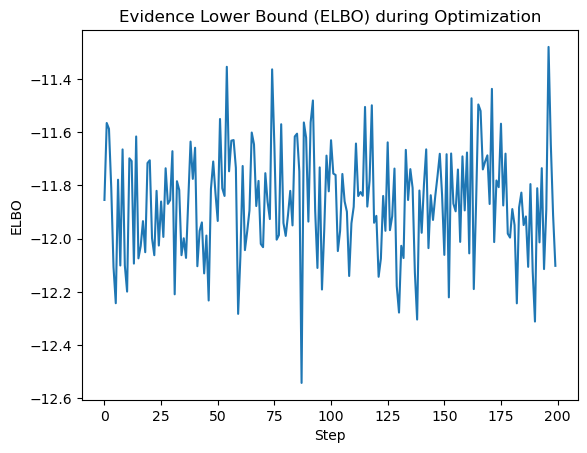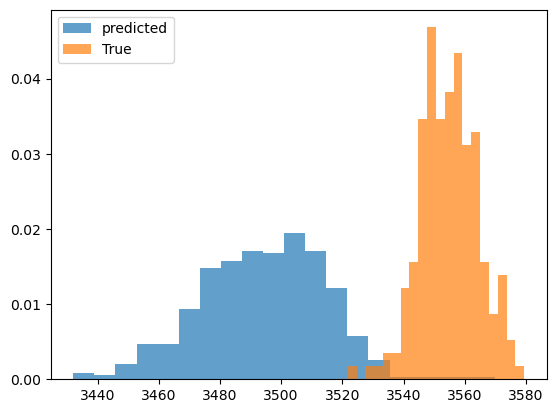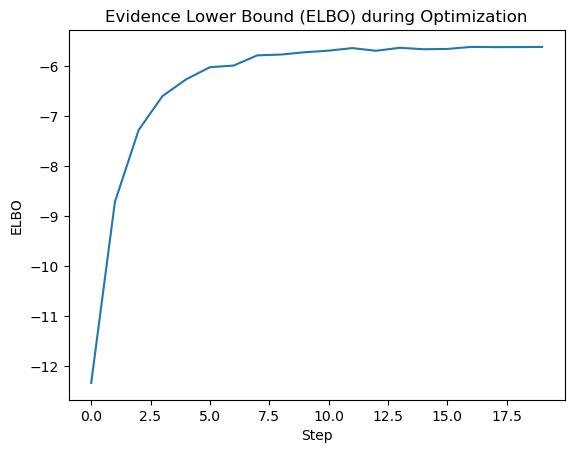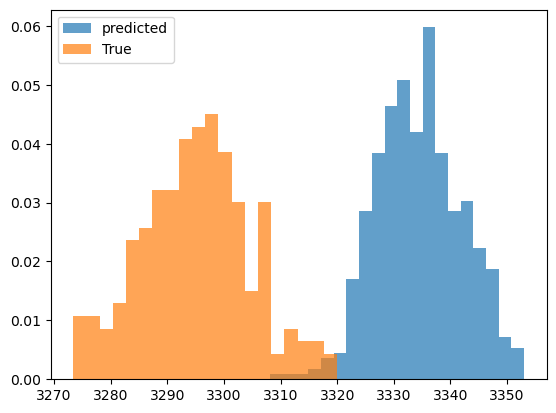# Variational Inference using SVI not converging

Hi, I am stuck with a seemingly simple problem. I have a mathematical model composed of various non-linear sums of gaussian distributions (x1, x2, x3, x4).

y = A*x1 + B*(torch.exp(-x2 / C)) + C*x3 + torch.log10(x4)

x1 and x2 are known variables, x3 and x4 have gaussian distribution with a fixed mean and std. I am using the simulated y as my observations and trying to infer the distribution of the latent variables x3 and x4.

Unfortunately, I am not able to infer the distribution of the ground truth. ELBO doesn’t seem to be maximizing no matter what I try. I tried different learning rates, changed the mean and std of the latent variables in the model and guide to be close to the true values, but it seems to do a random walk around the parameters I have specified in the model and fail to converge. I also tried using AutoDiagonalNormal, it seems to do a little better, but the posterior values seem to converge to some local minima far from my ground truth.
I must be doing something horribly wrong. If the only way for me to infer the latent variable is by known the prior to be very close to the truth, then I could simply use my model and try varying the unknown parameters until it matches with the distribution. My current code isn’t even able to get me to the ballpark.

I would appreciate if you could suggest ways to make the model robust and help with optimal strategy to select the model parameters.

## Define the mathematical model

def model(y,x1,x2):
x3 = pyro.sample("x3", dist.Normal(200, 100))
x4 = pyro.sample("x4", dist.Normal(120, 90))
# Use the mathematical model to compute the expected output
expected_output = A*x1 + B*(torch.exp(-x2 / C))  + C*x3 + torch.log10(x4)
# Likelihood of the observed output
pyro.sample("y", dist.Normal(expected_output, 20.0), obs=y)

## Define the updated guide with Normal distributions for x3 and x4
def guide(y,x1,x2):
x3_mean_guide = 150.0
x3_std_guide = 10.0
x4_mean_guide = 120.0
x4_std_guide = 25.0
# Sample x3 and x4 from the guide as Normal
x3 = pyro.sample("x3", dist.Normal(x3_mean_guide, x3_std_guide))
x4 = pyro.sample("x4", dist.Normal(x4_mean_guide, x4_std_guide))
return {"x3": x3, "x4": x4}

## Generate synthetic data
A = 50.0
B = 150.
C = 2.0
D = 10.0

### Specify known information about x1 and x2 (mean and std deviation)
x1_mean = 60.0
x1_stddev = 5.0
x2_mean = 10.0
x2_stddev = 2.0

x3_mean_true = 180.0
x3_stddev_true = 5.0

x4_mean_true = 80.0
x4_stddev_true = 25.0

x1 = pyro.sample("x1", dist.Normal(x1_mean, x1_stddev))
x2 = pyro.sample("x2", dist.Normal(x2_mean, x2_stddev))

## Simulated data
true_x3 = dist.Normal(x3_mean_true, x3_stddev_true).sample((200,))
true_x4 = dist.Normal(x4_mean_true, x4_stddev_true).sample((200,))

observed_output = A*x1 + B*(torch.exp(-x2 / C))  + C*true_x3 + torch.log10(true_x4)

## Setup SVI for variational inference
pyro.clear_param_store()
#guide = AutoDiagonalNormal(model)

adam_params = {"lr": .01, "betas": (0.95, 0.999)}
svi = SVI(model, guide, optimizer, loss=TraceGraph_ELBO())
elbo_values = [ ]
num_steps = 30
for step in range(num_steps):
loss = 0
for val in observed_output:
loss += svi.step(val, x1, x2)
elbo_values.append(-loss / len(observed_output))
print(step, loss)
## Extract the learned distribution of x3 and x4 from the guide
samples = [ ]
num_samples = 500
for _ in range(num_samples):
posterior = guide(observed_output,x1,x2)
x3_sample = posterior["x3"].item()
x4_sample = posterior["x4"].item()
samples.append((x3_sample, x4_sample))

## Print the mean and standard deviation of the inferred distributions
x3_samples = [x for x in samples]
x4_samples = [x for x in samples]

print("x3 mean:", torch.mean(torch.tensor(x3_samples)))
print("x3 std dev:", torch.std(torch.tensor(x3_samples)))
print("x4 mean:", torch.mean(torch.tensor(x4_samples)))
print("x4 std dev:", torch.std(torch.tensor(x4_samples)))

## Visualize the Data
### Convert true values to numpy arrays
true_x3_value = true_x3.numpy()
true_x4_value = true_x4.numpy()
predicted_output=A*x1 + B*(torch.exp(-x2 / C))  + C*torch.tensor(x3_samples) + torch.log10(torch.tensor(x4_samples))

plt.figure()
plt.hist(predicted_output.detach().numpy(), bins=20,alpha=0.7,label='predicted',density=True)
plt.hist(observed_output.detach().numpy(), bins=20,alpha=0.7,label='True',density=True)
plt.legend()

### Visualize the true values vs the inferred distributions
plt.figure(figsize=(12, 4))
plt.subplot(1, 2, 1)
plt.hist(x3_samples, bins=30, density=True, alpha=0.7, color='green', label='Inferred Distribution')
plt.hist(true_x3.detach().numpy(), bins=30, density=True, alpha=0.7, color='blue', label='True Distribution')
plt.xlabel('x3 values')
plt.ylabel('Probability Density')
plt.legend()

plt.subplot(1, 2, 2)
plt.hist(x4_samples, bins=30, density=True, alpha=0.7, color='green', label='Inferred Distribution')
plt.hist(true_x4.detach().numpy(), bins=30, density=True, alpha=0.7, color='blue', label='True Distribution')
plt.title('Inferred Distribution of log(x4) vs True log(x4)')
plt.xlabel('x4 values')
plt.ylabel('Probability Density')
plt.legend()

plt.tight_layout()
plt.show()

# Plot the ELBO values
plt.plot(elbo_values)
plt.xlabel('Step')
plt.ylabel('ELBO')
plt.title('Evidence Lower Bound (ELBO) during Optimization')
plt.show()



Here is the ELBOInferred vs Truth Latent Variables:

Inferred vs Truth Output Variables:Here is an example if I use AutoDiagonalNormal as a guide. The ELBO is ‘converging’ to some local minima.pyro.clear_param_store()
guide = AutoDiagonalNormal(model)
optimizer = torch.optim.SGD
scheduler = pyro.optim.ExponentialLR({'optimizer': optimizer, 'optim_args': {'lr': 0.01}, 'gamma': 0.1})
svi = SVI(model, guide, scheduler, loss=TraceGraph_ELBO())


I went through this tutorial without any luck. SVI Part IV: Tips and Tricks — Pyro Tutorials 1.8.6 documentation

didn’t look at your code in detail but i’d suggest taking another look at the tutorial, in particular

1. Pay attention to scales (basically choose units so that e.g. x3 and x4 are order unity) <== especially this one
1. If you are having trouble constructing a custom guide, use an AutoGuide
1. Parameter initialization matters: initialize guide distributions to have low variance

I tried using AutoGuide (AutoDiagonalNormal) it didn’t help.
Could you please elaborate on what normalization means in this context? My observed data (in my case simulated y) is a function of the original distribution for x3 and x4. Wouldn’t normalizing the latent variables in the model and guide make it infeasible for the model to find the right parameters? I am not understanding something basic here. I would appreciate it if you could provide some more feedback. Thanks!

e.g. you can make the replacement

x3_prime = pyro.sample("x3_prime", dist.Normal(2, 1))
x3 = pyro.deterministic("x3", 100 * x3_prime)


which doesn’t change the underlying model

num_steps = 30

also training for 30 steps is way too little. try 1000 or more

Thanks for getting back to me. This didn’t help. I have the training steps to be a lot longer, but it basically seems to get stuck at a local minima.

Normally, how would you pick the mean and std of the model latent variables? Let’s say my prior data tell me that the latent variables have narrow distribution, but I don’t have an exact information on the mean values. If I specify my model to have a narrow std with a mean value that might not be closer to the exact value (in the case of simulated data where I know the latent variable), the model fails to find the latent variable. If I specify the std to be a large value so the optimization will search through a larger parameter space, then my predicted latent value will also have a large standard deviation.

I would really appreciate if you could play around with the code and show what tuning is needed to make a good posteriori prediction. I am stuck at this for a few days, I would really appreciate any help.

Your guide is not learnable. x3_mean_guide and others should be pyro.params:

x3_mean_guide = pyro.param("x3_mean_guide", torch.tensor(150.0))
# same for others
# make sure that constraints are correct
...


Thanks @ordabayev, fixing the guide to tensor made the model learnable. I realize that there are multiple equivalent distributions of the latent variables that will result in very similar outcome, thus my predicted vs actual parameters don’t match well, makes sense. However, if I change the input parameters, the model still has hard time converging to the actual value.

I still have a general question. In my example, how would I specify the guide mean and std values for the latent variables. How about the mean, std and expected distribution std for the model?

As you train can you also record values of pyro.params and plot them. That will help to directly see how the posterior distribution is changing over iterations.

+x3_means = []
+...  # other guide params
for step in range(num_steps):
loss = 0
for val in observed_output:
loss += svi.step(val, x1, x2)
elbo_values.append(-loss / len(observed_output))
+    x3_means.append(pyro.param("x3_mean_guide").item())
+    ...
print(step, loss)


Also note that posterior distribution is affected by your prior and likelihood. For debugging purposes try to reduce likelihood noise (in fact in your simulation there is no likelihood noise at all):

pyro.sample("y", dist.Normal(expected_output, 1), obs=y)


Sorry, I don’t understand the question.

Here is what I don’t have a good intuition/understanding for. In my example, as I am simulating observed_output, I know what the mean and standard of x3 and x4 (in reality I would not know this as I would only have observed_output).
x3_mean_true = 180, x4_mean_true = 80
In order to find the latent variables (x3 and x4) based on the observed_output, I need to specify the distributions for x3 and x4 in the guide and model (which I know to be gaussian) along with a good guess for mean and std. Assuming I don’t have a good knowledge of the mean and std (which is the case in reality), how do I set the initial values for x3_mean_guide, x3_std_guide and x4_mean_guide, x4_std_guide? Similarly, for the model(), how do I specify the mean and std for x3 and x4, how about the std on the pyro.sample() ?

In the model you specify priors, which reflect your belief about the parameters before you have seen the data. Typically, if little know about the parameters then priors are set to wide distributions (with large std). On the other hand, if you have a tight prior (with small std) then it have larger effect on posterior (it will be more skewed to the prior).

For the guide it can be a trial and error or based on your intuition. In theory, initial guesses shouldn’t matter. In practice, bad initial guesses can make fitting slow or get the model stuck in local maxima. Same as in machine learning in general.

If I specify the std to be a small value  pyro.sample("y", dist.Normal(expected_output, 1), obs=y) then the predicted value is wacky

Also don’t use exponential scheduler with gamma=0.1 because your lr will drop very fast.

Thanks for all the comments. I am very close to get this example working.
Here is my final code along with the output. Sorry the values have changed by a bit as I played around with the model and parameters. No matter what I try I am not able to get x3 match with the true value. I still don’t have a good understanding on setting the values in the model. For instance, if I increase the std of x3, my elbo diverges.

import torch
import pyro
import pyro.distributions as dist
from pyro.infer import SVI, Trace_ELBO
import matplotlib.pyplot as plt
import numpy as np

# Define the mathematical model
def model(y,x1,x2):
# Prior distributions for the latent variables x3, x4
x3 = pyro.sample("x3", dist.Normal(100, 20))
x4 = pyro.sample("x4", dist.Normal(400, 40))
# Use the mathematical model to compute the expected output
expected_output = A*x1 + B*(torch.exp(-x2 / C))  + C*torch.exp(-x3) + D*x4
pyro.sample("y", dist.Normal(expected_output, 300.0), obs=y)

# Define the updated guide with LogNormal distributions for x3 and x4
def guide(y,x1,x2):
x3_mean_guide = 110.0
x3_std_guide = 10.0
x4_mean_guide = 300.0
x4_std_guide = 25.0
# Sample x3 and x4 from the guide as Normal
x3_mean_guide = pyro.param("x3_mean_guide", torch.tensor(x3_mean_guide))
x3_std_guide = pyro.param("x3_std_guide", torch.tensor(x3_std_guide))
x3 = pyro.sample("x3", dist.Normal(x3_mean_guide, x3_std_guide))

x4_mean_guide = pyro.param("x4_mean_guide", torch.tensor(x4_mean_guide))
x4_std_guide = pyro.param("x4_std_guide", torch.tensor(x4_std_guide))
x4 = pyro.sample("x4", dist.Normal(x4_mean_guide, x4_std_guide))

return {"x3": x3, "x4": x4}

# Generate synthetic data
A = 50.0
B = 150.
C = 20.0
D = 10.0

# Specify known information about x1 (mean and std deviation)
x1_mean = 60.0
x1_stddev = 5.0
x2_mean = 10.0
x2_stddev = 2.0

x3_mean_true = 80.0
x3_stddev_true = 5.0

x4_mean_true = 380.0
x4_stddev_true = 25.0

x1 = pyro.sample("x1", dist.Normal(x1_mean, x1_stddev))
x2 = pyro.sample("x2", dist.Normal(x2_mean, x2_stddev))

## Simulated data
true_x3 = dist.Normal(x3_mean_true, x3_stddev_true).sample((200,))
true_x4 = dist.Normal(x4_mean_true, x4_stddev_true).sample((200,))
observed_output = A*x1 + B*(torch.exp(-x2 / C))  + C*torch.exp(-true_x3) + D*true_x4

# Setup SVI
pyro.clear_param_store()

adam_params = {"lr": .005, "betas": (0.95, 0.999)}
svi = SVI(model, guide, optimizer, loss=Trace_ELBO())

elbo_values = []
x3_means = []
x3_std = []
x4_means = []
x4_std = []
num_steps = 400
for step in range(num_steps):
loss = 0
for val in observed_output:
loss += svi.step(val, x1, x2)
elbo_values.append(-loss / len(observed_output))
x3_means.append(pyro.param("x3_mean_guide").item())
x3_std.append(pyro.param("x3_std_guide").item())
x4_means.append(pyro.param("x4_mean_guide").item())
x4_std.append(pyro.param("x4_std_guide").item())
print(step, loss)

# Extract the learned distribution of x3 and x4 from the guide
samples = []
num_samples = 500
for _ in range(num_samples):
posterior = guide(observed_output,x1,x2)
x3_sample = posterior["x3"].item()
x4_sample = posterior["x4"].item()
samples.append((x3_sample, x4_sample))

# Print the mean and standard deviation of the inferred distributions
x3_samples = [x for x in samples]
x4_samples = [x for x in samples]

print("x3 mean:", torch.mean(torch.tensor(x3_samples)))
print("x3 std dev:", torch.std(torch.tensor(x3_samples)))
print("x4 mean:", torch.mean(torch.tensor(x4_samples)))
print("x4 std dev:", torch.std(torch.tensor(x4_samples)))

# Plots of the variable
plt.figure(figsize=(8,3))

plt.subplot(1,2,1)
plt.plot(x3_means, label='Predicted')
plt.fill_between(np.arange(len(x3_means)),np.array(x3_means)-np.array(x3_std),np.array(x3_means)+np.array(x3_std), alpha=0.2)
plt.plot(np.ones(len(x3_means))*x3_mean_true, label='True', color='b')
plt.fill_between(np.arange(len(x3_means)),np.array(x3_mean_true)-np.array(x3_stddev_true),np.array(x3_mean_true)+np.array(x3_stddev_true), alpha=0.2, color = 'b')
plt.xlabel('Iteration', fontsize=14)
plt.ylabel(r'X3 Value: $\mu \pm 1\sigma$', fontsize=14)
plt.legend()

plt.subplot(1,2,2)
plt.plot(x4_means, label='Predicted')
plt.fill_between(np.arange(len(x4_means)),np.array(x4_means)-np.array(x4_std),np.array(x4_means)+np.array(x4_std), alpha=0.2)
plt.plot(np.ones(len(x4_means))*x4_mean_true, label='True', color='b')
plt.fill_between(np.arange(len(x4_means)),np.array(x4_mean_true)-np.array(x4_stddev_true),np.array(x4_mean_true)+np.array(x4_stddev_true), alpha=0.2, color = 'b')

plt.xlabel('Iteration', fontsize=14)
plt.ylabel(r'X4 Value: $\mu \pm 1\sigma$', fontsize=14)
plt.tight_layout()
plt.legend()

# Convert true values to numpy arrays
true_x3_value = true_x3.numpy()
true_x4_value = true_x4.numpy()

# Visualize the true values vs the inferred distributions
plt.figure(figsize=(8, 3))
plt.subplot(1, 2, 1)
plt.hist(x3_samples, bins=30, density=True, alpha=0.7, color='green', label='Inferred Distribution')
plt.hist(true_x3.detach().numpy(), bins=30, density=True, alpha=0.7, color='blue', label='True Distribution')

plt.title('Inferred Distribution of log(x3) vs True log(x3)')
plt.xlabel('x3 values')
plt.ylabel('Probability Density')
plt.legend()

plt.subplot(1, 2, 2)
plt.hist(x4_samples, bins=30, density=True, alpha=0.7, color='green', label='Inferred Distribution')
plt.hist(true_x4.detach().numpy(), bins=30, density=True, alpha=0.7, color='blue', label='True Distribution')
plt.title('Inferred Distribution of log(x4) vs True log(x4)')
plt.xlabel('x4 values')
plt.ylabel('Probability Density')
plt.legend()

plt.tight_layout()
plt.show()

plt.figure(figsize=(8, 3))
plt.subplot(1, 2, 1)
# Plot the ELBO values
plt.plot(elbo_values)
plt.xlabel('Step')
plt.ylabel('ELBO')
plt.title('Evidence Lower Bound (ELBO) during Optimization')

predicted_value = A*x1 + B*(torch.exp(-x2 / C))  + C*torch.exp(-torch.tensor(x3_samples)) + D*torch.tensor(x4_samples)
predicted_value=predicted_value.detach().numpy()

plt.subplot(1, 2, 2)
plt.hist(predicted_value, label='predicted',alpha=0.5, density=True)
plt.hist(observed_output.detach().numpy(), label='training',alpha=0.5,density=True)
plt.legend()
plt.title('Truth vs Reconstructed')
plt.tight_layout()
plt.show()



Here are the outputs.

Your Truth vs Reconstructed histogram tells that the model has fit very well.

Now, your x3 posterior looks exactly like your prior. The reason must be that x3 has little effect on generating data, or more precisely, there is little you can tell about the x3 given your data so p(x3|data) = p(x3).

That makes sense! Are there other ways I can improve my inference method? How would I enforce that the ELBO will not diverge (I notice in some cases the ELBO diverges)

It probably depends case by case. Things to try I guess is to find better initial guesses and adjust learning rate.

I wanted to ask this again, sorry for too many responses.
should I have a much larger mean and std for the model and have a tighter mean and std for the guide? How would I strategies on setting the values of the latent variables’ distributions? Are there any tools that I can use to assign more optimal values if I don’t know what the mean and std of the latent variables are? Thanks

Choosing priors is a vast topic and there is no one solution fits all. There are many books written about it. Maybe you can start here: Prior probability - Wikipedia

This is also a complex topic and I can’t give an advice on it. Maybe there are other people on the forum that can help.

I am still struggling to make use out of this package, largely from the fact that I am having trouble setting appropriate bounds on the model and guide. It is a hit or miss on whether I can use the tool to predict the priori or not on my simulated data, that leaves me with very little confidence that I can use it in my problemI see in this example that the author uses HorseShoe prior that performs significantly better on converging.
Tutorial2 (robsalomone.com)

How would I adapt this in my model defined above?

def HorseshoeLR():
# ---- p(theta) -----------------------
tau = pyro.sample('tau', dist.HalfCauchy(scale=1.))
lam = pyro.sample('lambda', dist.HalfCauchy(scale=1.).expand([d]).to_event(1))

beta = sample('beta', Normal(loc=0. , scale=tau*lam).to_event(1) )
sigma = pyro.param("sigma", torch.tensor(1.),  constraint=dist.constraints.positive)

# ---- p(y|theta) ---------------------
with pyro.plate("data", X.shape):
y = pyro.sample('y', Normal(X @ beta.reshape(-1,1), sigma**2).to_event(1), obs = y_obs)

return y


Here is my attempt, but I run into nan issues trying to run the svi. how would I adapt to appropriately set the parameters so that they would be on the same ballpark as my model’s mean and std.

def model(y,x1,x2):
# ---- p(theta) -----------------------
tau = pyro.sample('tau', dist.HalfCauchy(scale=1.))
lam = pyro.sample('lambda', dist.HalfCauchy(scale=1.).expand().to_event(1))

beta = pyro.sample('beta', dist.Normal(loc=0. , scale=tau*lam).to_event(1) )
sigma = pyro.param("sigma", torch.tensor(1.),  constraint=dist.constraints.positive)
x3 = pyro.sample('x3', dist.Normal(beta.reshape(-1,1), sigma**2).to_event(1))

tau = pyro.sample('tau2', dist.HalfCauchy(scale=1.))
lam = pyro.sample('lambda2', dist.HalfCauchy(scale=1.).expand().to_event(1))

beta = pyro.sample('beta2', dist.Normal(loc=0. , scale=tau*lam).to_event(1) )
sigma = pyro.param("sigma2", torch.tensor(1.),  constraint=dist.constraints.positive)
x4 = pyro.sample('x4', dist.Normal(beta.reshape(-1,1), sigma**2).to_event(1))
expected_output = A*x1 + B*(torch.exp(-x2 / C))  + C*x3 + torch.log10(x4)

# ---- p(y|theta) ---------------------

y = pyro.sample('y', dist.Normal(expected_output, sigma**2).to_event(1), obs = y_obs)